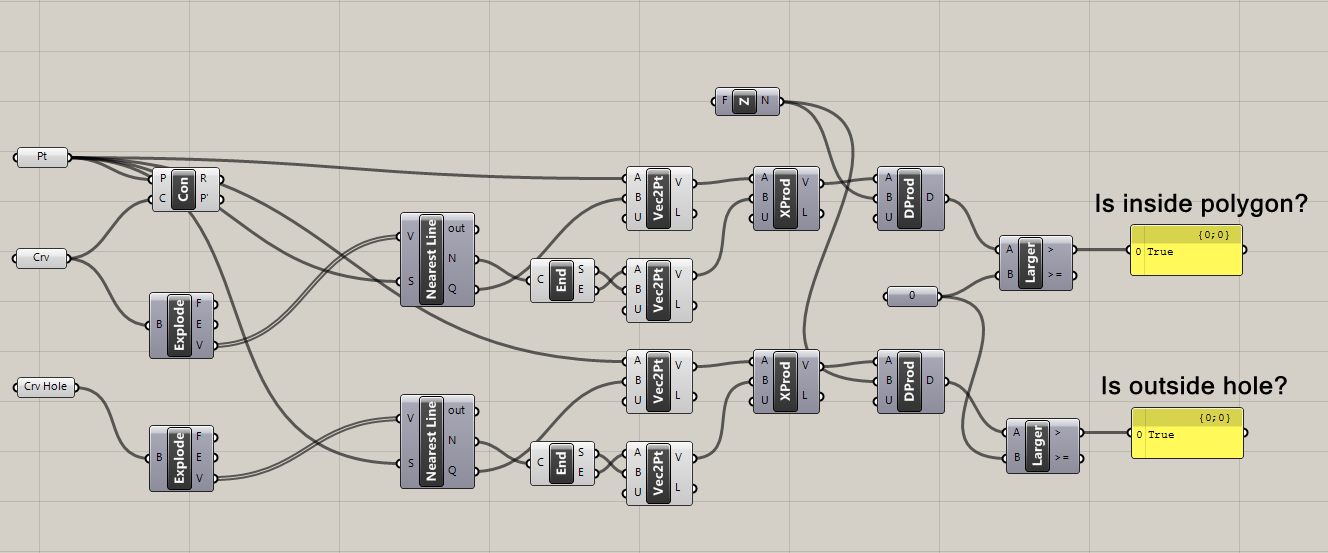# Where in the curve is the point?

Assuming there are two closed curves, one of them is created by points on a counter-clockwise direction, while the other one is created on a clockwise direction. Let this clockwise curve be a hole inside the counter-clockwise curve.

Suppose we have a point place elsewhere in the graph along with the aforementioned curves, and we need to know if the point lies inside the closed curves or not, what shall we do? Also, we need to know if the point is inside the hole or not.

I have created a somewhat graphical pseudonym using Rhino and Grasshopper to demonstrate this situation. Look at the image below to see the flow.By the way, the Con node has nothing to do with this flow so disregard it. Anyways, for your information, the Con mode, or the Containment node, checks the whereabouts of a point to a curve, and it returns one of the three values here (0 for outside, 1 for coincidence, 2 for inside). But this node does not recognize a closed curve being designated as a hole.

Exploding the curves give us three components: faces, edges and vertices. In this case, we only need vertices.

The two Nearest Line nodes are two same nodes I customized, and these nodes acquires the nearest segment by the point (N), and the closest point along that acquired line (Q). These two values are important for this process.

Get the endpoints of each segment, and produce a vector from those endpoints, thus

vector of the nearest line = endpoint (E) – startpoint (S)

We will also need to get the perpendicular vector with reference to the subject point, that is

perpendicular vector = point at (Q) – subject point

Get the cross product of these two vectors, and use this cross product to get the dot product of it with the normal vector (in this case, the Z node, or the Z-vector node).

Then we have to check if the dot product is greater than 0 or not. If the dot product is greater, we can say that the point is inside the closed curve.

On the contrary, when the point is outside the curve representing a hole, it returns true.

Tags: